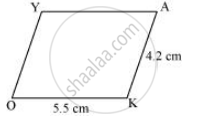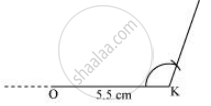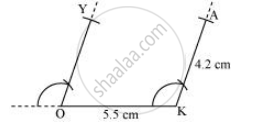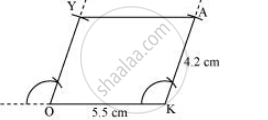# Draw the Following: a Parallelogram Okay Where Ok = 5.5 Cm and Ka = 4.2 Cm. - Mathematics

#### Question

Draw the following:

A parallelogram OKAY where OK = 5.5 cm and KA = 4.2 cm.

#### Solution

Opposite sides of a parallelogram are equal and parallel to each other. The given parallelogram OKAY can be drawn as follows.

1) A rough sketch of this parallelogram OKAY is drawn as follows.2) Draw a line segment OK of 5.5 cm and a ray at point K at a convenient angle.3) Draw a ray at point O parallel to the ray at K. As the vertices, A and Y, are 4.2 cm away from the vertices K and O respectively, cut line segments KA and OY, each of 4.2 cm, from these rays.4) Join Y to A.OKAY is the required parallelogram.

Is there an error in this question or solution?

#### APPEARS IN

NCERT Solution for Mathematics Textbook for Class 8 (2018 (Latest))
Chapter 4: Practical Geometry
Exercise 4.5 | 4 | Page no. 68

#### Video TutorialsVIEW ALL 

Draw the Following: a Parallelogram Okay Where Ok = 5.5 Cm and Ka = 4.2 Cm. Concept: Some Special Cases.